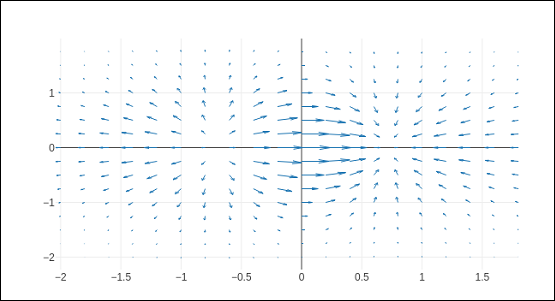# Plotly - Box Plot Violin Plot and Contour Plot

This chapter focusses on detail understanding about various plots including box plot, violin plot, contour plot and quiver plot. Initially, we will begin with the Box Plot follow.

## Box Plot

A box plot displays a summary of a set of data containing the minimum, first quartile, median, third quartile, and maximum. In a box plot, we draw a box from the first quartile to the third quartile. A vertical line goes through the box at the median. The lines extending vertically from the boxes indicating variability outside the upper and lower quartiles are called whiskers. Hence, box plot is also known as box and whisker plot. The whiskers go from each quartile to the minimum or maximum.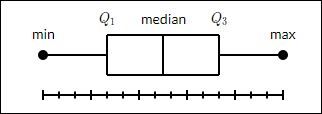To draw Box chart, we have to use go.Box() function. The data series can be assigned to x or y parameter. Accordingly, the box plot will be drawn horizontally or vertically. In following example, sales figures of a certain company in its various branches is converted in horizontal box plot. It shows the median of minimum and maximum value.

trace1 = go.Box(y = [1140,1460,489,594,502,508,370,200])
data = [trace1]
fig = go.Figure(data)
iplot(fig)


The output of the same will be as follows −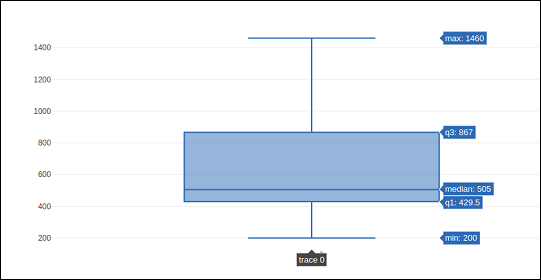The go.Box() function can be given various other parameters to control the appearance and behaviour of box plot. One such is boxmean parameter.

The boxmean parameter is set to true by default. As a result, the mean of the boxes' underlying distribution is drawn as a dashed line inside the boxes. If it is set to sd, the standard deviation of the distribution is also drawn.

The boxpoints parameter is by default equal to "outliers". Only the sample points lying outside the whiskers are shown. If "suspectedoutliers", the outlier points are shown and points either less than 4"Q1-3"Q3 or greater than 4"Q3-3"Q1 are highlighted. If "False", only the box(es) are shown with no sample points.

In the following example, the box trace is drawn with standard deviation and outlier points.

trc = go.Box(
y = [
0.75, 5.25, 5.5, 6, 6.2, 6.6, 6.80, 7.0, 7.2, 7.5, 7.5, 7.75, 8.15,
8.15, 8.65, 8.93, 9.2, 9.5, 10, 10.25, 11.5, 12, 16, 20.90, 22.3, 23.25
],
boxpoints = 'suspectedoutliers', boxmean = 'sd'
)
data = [trc]
fig = go.Figure(data)
iplot(fig)


The output of the same is stated below −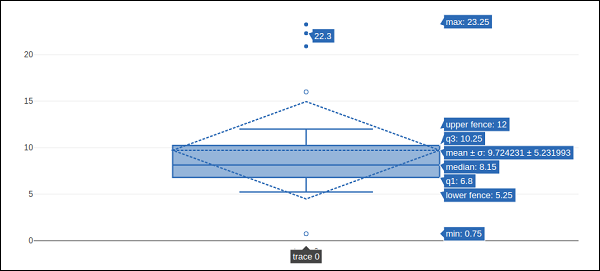## Violin Plot

Violin plots are similar to box plots, except that they also show the probability density of the data at different values. Violin plots will include a marker for the median of the data and a box indicating the interquartile range, as in standard box plots. Overlaid on this box plot is a kernel density estimation. Like box plots, violin plots are used to represent comparison of a variable distribution (or sample distribution) across different "categories".

A violin plot is more informative than a plain box plot. In fact, while a box plot only shows summary statistics such as mean/median and interquartile ranges, the violin plot shows the full distribution of the data.

Violin trace object is returned by go.Violin() function in graph_objects module. In order to display underlying box plot, the boxplot_visible attribute is set to True. Similarly, by setting meanline_visible property to true, a line corresponding to the sample's mean is shown inside the violins.

Following example demonstrates how Violin plot is displayed using plotly’s functionality.

import numpy as np
np.random.seed(10)
c1 = np.random.normal(100, 10, 200)
c2 = np.random.normal(80, 30, 200)
trace1 = go.Violin(y = c1, meanline_visible = True)
trace2 = go.Violin(y = c2, box_visible = True)
data = [trace1, trace2]
fig = go.Figure(data = data)
iplot(fig)


The output is as follows −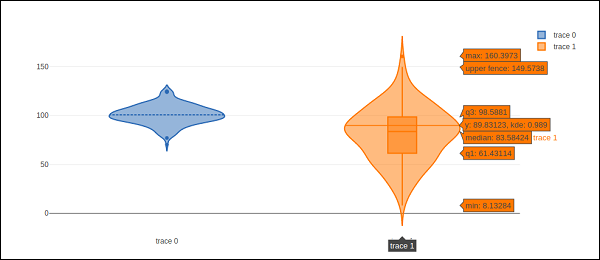## Contour plot

A 2D contour plot shows the contour lines of a 2D numerical array z, i.e. interpolated lines of isovalues of z. A contour line of a function of two variables is a curve along which the function has a constant value, so that the curve joins points of equal value.

A contour plot is appropriate if you want to see how some value Z changes as a function of two inputs, X and Y such that Z = f(X,Y). A contour line or isoline of a function of two variables is a curve along which the function has a constant value.

The independent variables x and y are usually restricted to a regular grid called meshgrid. The numpy.meshgrid creates a rectangular grid out of an array of x values and an array of y values.

Let us first create data values for x, y and z using linspace() function from Numpy library. We create a meshgrid from x and y values and obtain z array consisting of square root of x2+y2

We have go.Contour() function in graph_objects module which takes x,y and z attributes. Following code snippet displays contour plot of x, y and z values computed as above.

import numpy as np
xlist = np.linspace(-3.0, 3.0, 100)
ylist = np.linspace(-3.0, 3.0, 100)
X, Y = np.meshgrid(xlist, ylist)
Z = np.sqrt(X**2 + Y**2)
trace = go.Contour(x = xlist, y = ylist, z = Z)
data = [trace]
fig = go.Figure(data)
iplot(fig)


The output is as follows −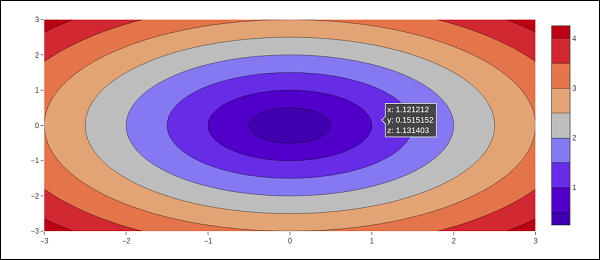The contour plot can be customized by one or more of following parameters −

• Transpose (boolean) − Transposes the z data.

If xtype (or ytype) equals "array", x/y coordinates are given by "x"/"y". If "scaled", x coordinates are given by "x0" and "dx".

• The connectgaps parameter determines whether or not gaps in the z data are filled in.

• Default value of ncontours parameter is 15. The actual number of contours will be chosen automatically to be less than or equal to the value of ncontours. Has an effect only if autocontour is "True".

Contours type is by default: "levels" so the data is represented as a contour plot with multiple levels displayed. If constrain, the data is represented as constraints with the invalid region shaded as specified by the operation and value parameters.

showlines − Determines whether or not the contour lines are drawn.

zauto is True by default and determines whether or not the color domain is computed with respect to the input data (here in z) or the bounds set in zmin and zmax Defaults to False when zmin and zmax are set by the user.

## Quiver plot

Quiver plot is also known as velocity plot. It displays velocity vectors as arrows with components (u,v) at the points (x,y). In order to draw Quiver plot, we will use create_quiver() function defined in figure_factory module in Plotly.

Plotly's Python API contains a figure factory module which includes many wrapper functions that create unique chart types that are not yet included in plotly.js, Plotly's open-source graphing library.

The create_quiver() function accepts following parameters −

• x − x coordinates of the arrow locations

• y − y coordinates of the arrow locations

• u − x components of the arrow vectors

• v − y components of the arrow vectors

• scale − scales size of the arrows

• arrow_scale − length of arrowhead.

• angle − angle of arrowhead.

Following code renders a simple quiver plot in Jupyter notebook −

import plotly.figure_factory as ff
import numpy as np
x,y = np.meshgrid(np.arange(-2, 2, .2), np.arange(-2, 2, .25))
z = x*np.exp(-x**2 - y**2)
v, u = np.gradient(z, .2, .2)

# Create quiver figure
fig = ff.create_quiver(x, y, u, v,
scale = .25, arrow_scale = .4,
name = 'quiver', line = dict(width = 1))
iplot(fig)


Output of the code is as follows −